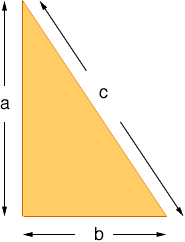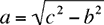Quandaries and Queries Who is asking: Student Level: Middle Question: If I know the base and the slanted side of a right triangle, how do I figure out the height? Hi Bill, You can use Pythagoras' Theorem. If a right triangle has side base of length b, height a and the slant side (called the hypotenuse) has length c then a2 + b2 = c2Thus if you know b and c thenPenny Go to Math Central